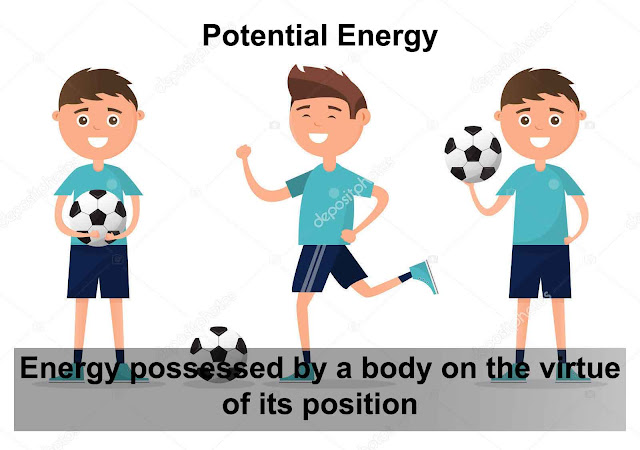# What is Potential Energy [Definition, Formula, Examples]

Hello friends, talking about potential energy (P.E), we already know that our specific definition of potential energy is suggested by the scientists and your teacher is that, It is nothing but an energy possessed by a body on the virtue of its position. One thing to look carefully is that here scientists mention that virtue of its position.

## What is Potential Energy?

This means that body is having energy but it totally based upon the position where it is lying.

• Learn and remember,  If it is lying on ground then one can easily say that the P.E energy is zero.

• If you just lift it up (maybe an object) to certain height then it means(we say) that body has increased its P.E energy to a certain value.

Making the above explanation more clear, we define Potential energy by illustration.  let's take ball as an example. Let's we lift it up firstly, at a 5 meter height. Later, we lift up to 10 meter height. Now things to consider and learn.

• In Both of the cases, the position of ball is not with the ground it is above ground. So, P.E is not Zero. It will be zero only if it lying on ground.(take ground as a reference point).

• Secondly, the energy possessed by a body on the basis of its position. It means that if a body is at the higher height then we have to predict that it is carrying more P.E as we see that a ball at 10 metre height has more potential energy and a ball at a 5 metre height has energy half of the first case.

## Formula of Potential Energy

ok, Now we look at the formula for potential energy. P.E energy is basically energy. So, we can say p is equals to mgh.

P.E= mgh

It simply means that the energy possessed by a body is totally based upon the mass of an object, gravity and height.

If we take two balls having equal mass. let's take an example 2 kg. and the gravity g is constant with the value of 9.8 metre per second square. So, one can easily predict that P.E energy is totally rely on the height as we discussed earlier.

What's more,

•  One can easily say that potential energy is directly proportional to height greater the height more will be the P.E energy.
P.E=(direcly proportional)Height

• Units of P.E energy is joules.
• It's having units equal to kinetic energy

Now look at the numerical. It will helps you to to identify the type of problem and allow you to ease in calculating the various numerical.

## Examples

• Derive an expression for the potential energy of the body. Calculate P.E of body of mass 10Kg at a height of 10m.
• P.E energy of a body of mass = m Kg
•  at a height = h m from the ground.
• Gravitational force of attraction on the body = mgN
• In order to lift this body A to B at h m above the ground.
• In order to lift this body with a constant velocity, force applied = mg N
•  Distance moved by force = h m
• Work done in lifting the body from a to B = Force × Distance = mg × h = mgh
• Energy spent in lifting the body to height ‘h’.

• As energy cannot be destroyed, this energy gets stored in the body as its potential energy
• m = 10 Kg
• g = 10 m/s2
• h = 10 m
• P. E = mgh = 10×10×10 = 1000 JoulesThanks for reading What is Potential Energy [Definition, Formula, Examples]

Previous
« Prev Post